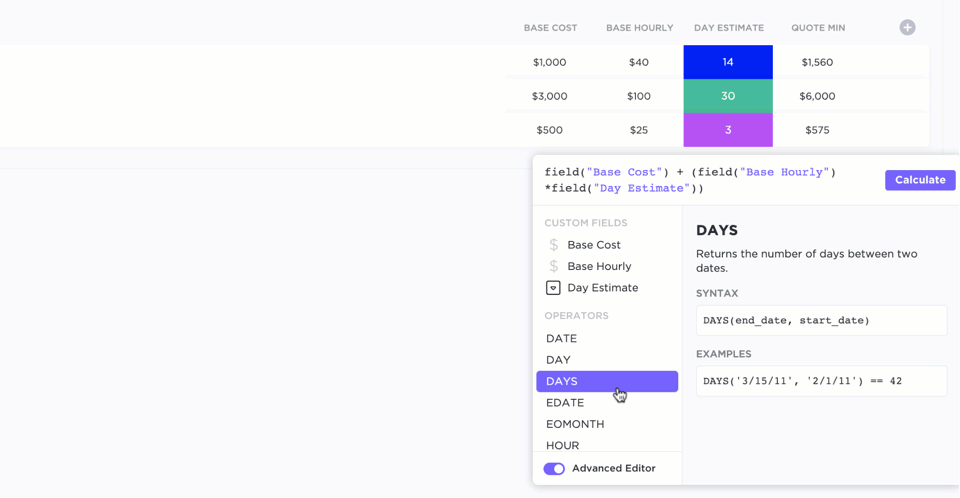# Calculate using Formula Fields

Formula Fields allow you to make calculations between numeric, date, and time fields on tasks using simple or advanced Formulas. They can be applied at any level of your Workspace Hierarchy, but they are not transferable to other locations.

Formula options automatically calculate the entire column. Editing a Formula in one task edits the Formula for the entire column.

# What you'll need

• 60 uses of Custom Fields, including Formula Fields, are available on the Free Forever Plan.
• Unlimited uses are available on all paid plans.
• Individual Custom Fields can be hidden from guests.
• Guests with edit or full permissions can edit existing Custom Fields but cannot create new ones.

# Create a Formula Field

To create a Formula Field in List view:

1. Scroll all the way right and click the + icon.
2. Select Formula.
3. Name the Formula Field.

# Supported fields

With over 70 different functions, Formulas can calculate data using the following fields:

• Number Custom Fields
• Currency Custom Fields
• Dropdown Custom Fields that only use numbers
• Date Custom Fields
• Start Date
• Due Date
• Date Created
• Date Started
• Date Updated
• Date Closed
• Time Tracked
• Time Tracked is in hours.
• Time Estimated
• Time Estimated is in hours.

# Simple Formulas

Simple Formulas are meant for quick addition, subtraction, division, and multiplication.

To create a simple Formula:

2. Select a value from the first dropdown.
3. Select an operator:
• Subtract
• Multiply
• Divide
4. Select a value from the second dropdown.
5. Click Calculate.

Advanced Formulas allow for more complex and flexible calculations.

2. Toggle on the Advanced Editor.
3. Search through the available Custom Fields and operators.
4. Write a valid Formula in the text field at the top.
5. Click Calculate.## Real-world examples

You can use advanced formulas to add or subtract days, show percent signs, round decimal places, and more! You can use advanced formulas to add or subtract days, show percent signs, round decimal places, and more!

 Desired outcome Method Example formula Add a specific number of days to the date field. Use the DATE function and apply basic mathematical expressions for YEAR(), MONTH(), OR DAY(). For example, to add seven days to a date: `DATE(YEAR(field("Due date")),MONTH(field("Due date")),DAY(field("Due date"))+7)` Subtract a specific number of days from the date field. Use the DATE function and apply basic mathematical expressions for YEAR(), MONTH(), OR DAY(). For example, to subtract five days from a date: `DATE(YEAR(DAYS(field("Due date"), 5)), MONTH(DAYS(field("Due date"), 5)), DAY(DAYS(field("Due date"), 5)))` Calculate the difference between two dates. Use the DAYS function to accomplish this. The following format is used for this formula: `DAYS(end_date, start_date)` To calculate the difference between a task's start and due date: `DAYS(field("Due date"),field("Start date"))` Calculate a percentage from a Custom Field. Instead of decimals, use percentages in the formula. For example: `field("Number Field")*10%` Color-code results. Use a formula to color-code results with emojis. For example: • If the age of a task is 10 days or less, add a green indicator. • If the age of a task is 20 days or less, add a yellow indicator. • If the age of a task is over 20 days, add a red indicator. The following formula would achieve the intended result: `IF(DAYS(TODAY(), field("Date created"))<11, "🟢", IF(DAYS(TODAY(), field("Date created"))<21, "🟡","🔴"))` Add a percent sign. Use the CONCATENATE function to add a percent sign. There are two ways to add a percent sign: • Use the CONCATENATE function: `CONCATENATE((field("Number 1")*field("Number 2")),"%")` • Use &"%"to save time: `(field("Number 1")*field("Number 2"))&"%"` Field names are case-sensitive. Round decimal places on results. Use the ROUND function to round results after using basic functions. The following format is used for this formula: `ROUND(value, [places])` To add two number fields and round the result to the hundredth decimal place, use the following formula: `ROUND((field("Number 1") + field("Number 2")),2)` Field names are case-sensitive.

Updated

<% if (previousArticle || nextArticle) { %>
<% if (previousArticle) { %>
<% if (previousTitle) { %>

#### <%= previousTitle %>

<% } %> <%= previousArticle.title %>
<% } %> <% if (nextArticle) { %>
<% if (nextTitle) { %>

#### <%= nextTitle %>

<% } %> <%= nextArticle.title %>
<% } %>
<% } %>
<% if (items.length > 1) { %>